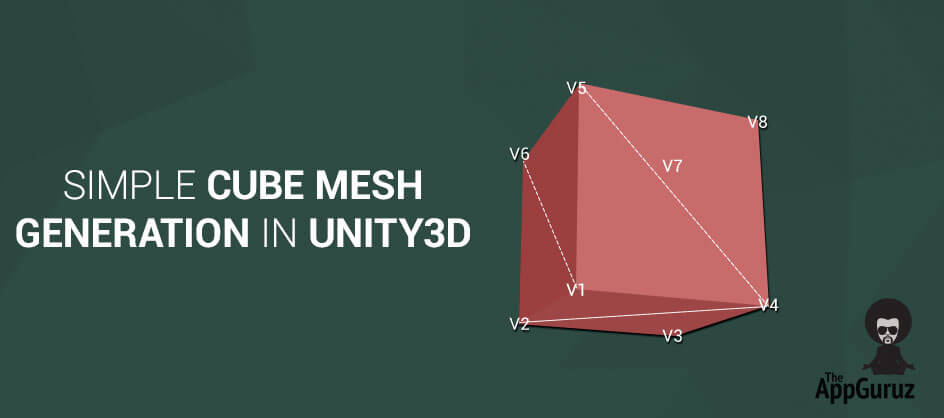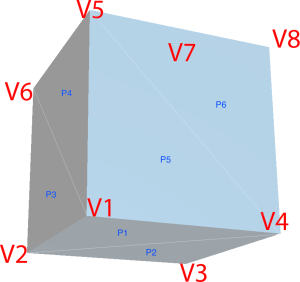## Objective

Main objective of this blog post is to give you an idea about Simple Cube Mesh Generation in Unity3D

Mesh:
A mesh is a collection of 3D coordinates (vertices) grouped together in triangular arrays to form the 3D shape. If you create a new cube object in a Unity3D project, and select it in the Hierarchy pane and check the Inspector panel, you can see that there is a component called Cube (Mesh Filter) that has a property called Mesh with the value Cube.

If you change the value of Cube, to say, Sphere, you’ll see that the object is now changed to a sphere. This is because by changing the mesh we have changed the 3D coordinates that dictate the shape of the object. Unity3D provides us a number of methods to manipulate these mesh objects dynamically, this is very powerful because it means we don’t have to rely on our modeling tools to create or manipulate 3D shapes.

## Step 1 Mesh Methods And Properties

### 1.1 The Vertices property

The vertices property is an array of Vector3D items that sets each vertex and defines the boundaries of the mesh.

### 1.2 The UV Property

The UV property is an array of Vector2D items that sets how the material is to be applied onto the mesh.

### 1.3 The Triangles Property

The triangles property is an array of integers, wherein each three integers in sequence represent a relationship of three vertices forming a triangle. So a single triangle object between the first, second and the third vertex items in the vertices array would exist in the triangles array as {0, 1, 2}.

A simple square shape would exist in the array with another three integers {0, 1, 2, 1, 2, 3}.

As you can see the triangles array works in groups of three, each group referring to the vertex items in the vertices array.

This property is an array of integers that set the triangles (polys) or the relationship of the vertices to one another.

### 1.4 The Clear() Method

This method will clear the triangle and vertex data from the mesh.

### 1.5 The RecalculateBounds() Method

We have to call the RecalculateBounds method to make sure that the triangles from the vertex relationships are recalculated correctly after vertex coordinates have been changed.

### 1.6 The RecalculateNormals() Method

The Normals of the mesh are the faces of the 3D object that are actually rendered. By calling this method we instruct the engine to recalculate the normal of the mesh.

## Step 2 Custom size cube exampleImage of cube mesh containing vertices and polygon.

Let’s Learn Above Methods by Creating Custom Size Cube Mesh example

### 2.1 GetVertices

This method contains The Array of All the Vertices in the Cube Mesh.

``````privateVector3[] GetVertices ()
{
Vector3 vertice_0 = newVector3 (-cubeLength * .5f, -cubeWidth * .5f, cubeHeight * .5f);
Vector3 vertice_1 = newVector3 (cubeLength * .5f, -cubeWidth * .5f, cubeHeight * .5f);
Vector3 vertice_2 = newVector3 (cubeLength * .5f, -cubeWidth * .5f, -cubeHeight * .5f);
Vector3 vertice_3 = newVector3 (-cubeLength * .5f, -cubeWidth * .5f, -cubeHeight * .5f);
Vector3 vertice_4 = newVector3 (-cubeLength * .5f, cubeWidth * .5f, cubeHeight * .5f);
Vector3 vertice_5 = newVector3 (cubeLength * .5f, cubeWidth * .5f, cubeHeight * .5f);
Vector3 vertice_6 = newVector3 (cubeLength * 1.5f, cubeWidth * .5f, -cubeHeight * .5f);
Vector3 vertice_7 = newVector3 (-cubeLength * .5f, cubeWidth * .5f, -cubeHeight * .5f);
Vector3[] vertices = newVector3[]
{
// Bottom Polygon
vertice_0, vertice_1, vertice_2, vertice_0,
// Left Polygon
vertice_7, vertice_4, vertice_0, vertice_3,
// Front Polygon
vertice_4, vertice_5, vertice_1, vertice_0,
// Back Polygon
vertice_6, vertice_7, vertice_3, vertice_2,
// Right Polygon
vertice_5, vertice_6, vertice_2, vertice_1,
// Top Polygon
vertice_7, vertice_6, vertice_5, vertice_4
} ;

return vertices;
}``````

### 2.2 GetNormals

This method returns The Rendering Face Of Cube Polygons.

``````/// <summary>
/// The Cube Side Which Are Use when rendering Cube
/// </summary>
/// <returns>The normals.</returns>
privateVector3[] GetNormals ()
{
Vector3 up = Vector3.up;
Vector3 down = Vector3.down;
Vector3 front = Vector3.forward;
Vector3 back = Vector3.back;
Vector3 left = Vector3.left;
Vector3 right = Vector3.right;

Vector3[] normales = newVector3[]
{
// Bottom Side Render
down, down, down, down,

// LEFT Side Render
left, left, left, left,

// FRONT Side Render
front, front, front, front,

// BACK Side Render
back, back, back, back,

// RIGTH Side Render
right, right, right, right,

// UP Side Render
up, up, up, up
} ;

returnnormales;
}``````

### 2.3 GetUVsMap

UV mapping refers to the way in which each 3D surface is mapped on a 2D texture. Each vertex contains a set of UV coordinates, where (0.0, 0.0) refers to the lower left corner of the texture and (1.0, 1.0) refers to the upper right corner of the texture.

If the UV coordinates are outside the 0.0 to 1.0 range, the coordinates are either clamped or the texture is repeated (depending of the texture import setting in Unity).

``````privateVector2[] GetUVsMap ()
{
Vector2 _00_CORDINATES = newVector2 (0f, 0f);
Vector2 _10_CORDINATES = newVector2 (1f, 0f);
Vector2 _01_CORDINATES = newVector2 (0f, 1f);
Vector2 _11_CORDINATES = newVector2 (1f, 1f);
Vector2[] uvs = newVector2[]
{
// Bottom
_11_CORDINATES, _01_CORDINATES, _00_CORDINATES, _10_CORDINATES,
// Left
_11_CORDINATES, _01_CORDINATES, _00_CORDINATES, _10_CORDINATES,
// Front
_11_CORDINATES, _01_CORDINATES, _00_CORDINATES, _10_CORDINATES,
// Back
_11_CORDINATES, _01_CORDINATES, _00_CORDINATES, _10_CORDINATES,
// Right
_11_CORDINATES, _01_CORDINATES, _00_CORDINATES, _10_CORDINATES,
// Top
_11_CORDINATES, _01_CORDINATES, _00_CORDINATES, _10_CORDINATES,
} ;
returnuvs;
}``````

### 2.4 GetTriangles

Array is a list of triangles that contain indices in the vertex array. The size of the triangle array must always be a multiple of 3. Vertices can be shared by simply indexing it into the same vertex. If the mesh contains multiple sub meshes (materials) the triangle list will contain all triangles of all the sub meshes.

When you assign triangle array, subMeshCount is set to 1. If you want to have multiple sub meshes, use subMeshCount and SetTriangles.

``````privateint[] GetTriangles ()
{
int[] triangles = newint[]
{
// Cube Bottom Side Triangles
3, 1, 0,
3, 2, 1,
// Cube Left Side Triangles
3 + 4 * 1, 1 + 4 * 1, 0 + 4 * 1,
3 + 4 * 1, 2 + 4 * 1, 1 + 4 * 1,
// Cube Front Side Triangles
3 + 4 * 2, 1 + 4 * 2, 0 + 4 * 2,
3 + 4 * 2, 2 + 4 * 2, 1 + 4 * 2,
// Cube Back Side Triangles
3 + 4 * 3, 1 + 4 * 3, 0 + 4 * 3,
3 + 4 * 3, 2 + 4 * 3, 1 + 4 * 3,
// Cube Rigth Side Triangles
3 + 4 * 4, 1 + 4 * 4, 0 + 4 * 4,
3 + 4 * 4, 2 + 4 * 4, 1 + 4 * 4,
// Cube Top Side Triangles
3 + 4 * 5, 1 + 4 * 5, 0 + 4 * 5,
3 + 4 * 5, 2 + 4 * 5, 1 + 4 * 5,
} ;
return triangles;
}``````

### 2.5 GetCubeMesh

This method is used to get mesh from the filter. If it does’nt have then add a new component.

``````privateMeshGetCubeMesh ()
{
if (GetComponent () == null) {
Meshmesh = filter.mesh;
mesh.Clear ();
return mesh;
}  else {
}
}``````

### 2.6 MakeCube

This method contains the call to all the above function results of mesh for generating a procedural cube in unity.

``````voidMakeCube ()
{
cubeMesh = GetCubeMesh ();
cubeMesh.vertices = GetVertices ();
cubeMesh.normals = GetNormals ();
cubeMesh.uv = GetUVsMap ();
cubeMesh.triangles = GetTriangles ();
cubeMesh.RecalculateBounds ();
cubeMesh.RecalculateNormals ();
cubeMesh.Optimize ();
}``````

I hope you find this blog very interesting while working with Cube Mesh Generation in Unity3D. Let me know in comment if you have any questions regarding Unity3D. I will reply you ASAP.

Got an Idea of 3D Game Development? What are you still waiting for? Contact us now and see the Idea live soon. Our company has been named as one of the best 3D Game Development Company in India.I am 3D game developer with an aspiration of learning new technology and creating a bright future in Information Technology.